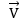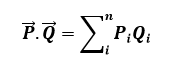# Vector algebra

Vector algebra is one of the essential topics of algebra. It studies the algebra of vector quantities. As we know, there are two types of physical quantities, scalars and vectors. The scalar quantity has only magnitude, whereas the vector quantity has both magnitude and direction.

Algebra is a hugely significant subject in Maths where we use universal symbols or letters to signify the quantities, numbers and variables. These symbols are later used in many expressions, equations and formulae, to perform algebraic operations. It has many branches.

In essence, vector algebra is an algebra where the essential elements normally denote vectors. We perform algebraic operations on vectors and vector spaces. This branch has rules and hypotheses based on the properties and behaviour of vectors. Here, we will learn various concepts based on the basics of vector algebra and some solved examples.

## Definition

A vector is an object which has both magnitude as well as direction. It is usually represented by an arrow which shows the direction(→) and its length shows the magnitude. The arrow which indicates the vector has an arrowhead and its opposite end is the tail. It is denoted as. The magnitude of the vector is represented as |V|. Two vectors are termed as equal if they have equal magnitudes and equal direction.

## Vector Algebra Operations

Just like in usual Algebra, we also perform arithmetic operations such as addition, subtraction, multiplication, in vectors. However, in the case of multiplication only, vectors have two terminologies, such as dot product and cross product, which we are going to learn here.

Let us consider there are two vectors P and Q, then the sum of these two vectors can be performed when the tail of vector Q meets with the head of vector A. And during this addition, the magnitude and direction of the vectors should not change. The vector addition follows two important laws, which are;

• Commutative Law: A + B = B + A
• Associative Law: A + (B + C) = (A + B) + C

### Subtraction Of Vectors

Here, the direction of other vectors is reversed and then the addition is performed on both the given vectors. If P and Q are the vectors, for which the subtraction method has to be performed, then we invert the direction of another vector say for Q, to make it -Q. Now, we need to add vector P and -Q. So here the direction of the vectors are opposite each other but the magnitude remains the same.

• P – Q = P + (-Q)

### Multiplication of Vectors

If k is a scalar quantity and it is multiplied by a vector A, then the scalar multiplication is given by kA. If k is positive then the direction of the vector kA is the same as vector A, but if the value of k is negative, then the direction of vector kP will be opposite to the direction of vector A. And the magnitude of the vector kP is given by |k|.

### Dot Product

The dot product is often called a scalar product. It is represented using a dot(.) between two vectors. Here, two coordinate vectors of equal length are multiplied in such a way that they result in a single number. So basically when we take the scalar product of two vectors the result is either a number of a scalar quantity. Suppose P and Q are two vectors, then the dot product for both the vectors is given by;

• P.Q = |P| |Q| cos θ

If P and Q are both in the same direction, i.e. θ = 0°, then;

• P.Q = |P| |Q|

If P and Q are both orthogonal, i.e. θ = 90°, then;

• P.Q = 0 [since cos 90° = 0]

In vector algebra, if two vectors are given as;

P = [P1,P2,P3,P4,….,Pn] and Q = [Q1,Q2,Q3,Q4,….,Qn]

Then their dot product is given by;

• P.Q = P1Q1+P2Q2+P3Q3+……….PnQn### Cross Product

A cross product is denoted by the multiplication sign(x) between two vectors. It is a binary vector operation, defined in a three-dimensional system. If P and Q are two independent vectors, then the result of the cross product of these two vectors (P x Q) is perpendicular to both the vectors and normal to the plane that contains both the vectors. It is represented by;

• P x Q = |P| |Q| sin θ

### Vector Algebra Example

Question: Find the dot product of vectors P(1, 3, -4) and Q(3,−5, 2).

Ans: As per the vector algebra definition of the dot product, we know;

P.Q = P1Q1+P2Q2+P3Q3+……….PnQn

Therefore,

P.Q = 1.3 + 3.(-5) + (-4).2

= 3 – 15 – 8

= -20

### What is Vector Algebra?

Vector algebra is defined as a type of algebra where the elements are in vector form and their algebraic operations follow the vector laws.

### What are the Laws of Vector Addition?

The two main laws of vector addition are:

Commutative Law: A + B = B + A
Associative Law: A + (B + C) = (A + B) + C

### What are the Types of Vector Multiplication?

There are two types of vector multiplication:

Dot Product: P • Q = |P| |Q| cos θ

Cross Product: P × Q = |P| |Q| sin θ